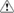Journal topic

# Comment on a paper

If you would like to submit an interactive comment (short comment, referee comment, editor comment, or author comment) for immediate non-peer-reviewed publication in the interactive discussion of a paper recently published in CPD, please locate this paper on the CPD papers in open discussion web page and follow the appropriate links there.

Short comments can be submitted by every registered member of the scientific community (free registration is accessible via the log-in link). They are restricted to a maximum length of 10 pages and have to be submitted within 8 weeks after publication of the discussion paper in CPD. For details see interactive public discussion.

## Activate LaTeX

If you want to use LaTeX commands in your comment, you need to "activate LaTeX commands" by clicking on the appropriate button just above the text input window.

## Template

The following template is a simple ASCII text file with a typical layout for interactive comments and some frequently used LaTeX commands. It can be viewed, edited, copied, and pasted into the text field of the comment submission form using any standard text editor: comment_example.txt.

## Line and paragraph breaksLaTeX ignores extra spacing between words. If you want to force a line break, please use a double backslash \\ in the appropriate place. For separating paragraphs, use two hard returns.

## Italic and bold text

Italic text may be created by putting the text into curly braces with a \it after the opening brace.Typing "{\it This is italic} and this is not." will produce "This is italic and this is not."

Remember to include the empty space between the \it and the rest of text. Bold face text is produced in a similar way using \bf.Typing "{\bf This is bold} and this is not." will produce "This is bold and this is not."

Again, remember to include the empty space between the \bf and the rest of text.

## Subscripts and superscripts

To create a subscript, type a dollar sign, an underscore, an open curly brace, the character(s) you want to be subscripted, a close curly brace, and another dollar sign.Typing "H$_{2}$SO$_{4}$" will produce "H2SO4" and typing "T$_{ice}$" will produce "Tice".

Creating a superscripts follows the same procedure with the difference that you need to put a caret sign instead of the underscore.Typing "cm$^{-3}$" will produce "cm-3" and typing "T$^{ice}$" will produce "Tice".

## Special characters

Some characters have a special function in LaTeX; if you want to use them as a normal character you need to put a backslash in front of them.\% \$\& \# \_ \{ \}In particular the percent sign is used to introduce commented text in LaTeX, so ALWAYS put the backslash in front of it or else some of your text will disappear. ## Greek symbols Greek symbols can be used by putting the special commands listed below between two dollar signs: \alpha, \beta, \gamma, \delta, \epsilon, \nu, \kapp,a \lambda, \mu, \pi, \omega, \sigma, etc.Typing "$\mu$m" will produce "µm". Similarly, upper-case Greek letters can be produced: \Gamma, \Delta, \Lambda, \Sigma, \Omega, \Theta, \Phi, etc. For a more complete list of symbols, please consult the two documents "Getting started with LaTeX" and "Not so Short Introduction to LaTeX2e". ## Mathematical symbols Some frequently used mathematical symbols are produced in the same way as Greek symbols: Typing$<$will produce <. Typing$>$will produce >. Typing$=$will produce =. Typing$\times$will produce ×. Typing$\pm$will produce ±. Typing$\sim$will produce ~. Typing$^\circ$will produce °. Typing$\rightarrow$will produce an arrow pointing to the right as frequently used in chemical reactions. ## Equations Simple equations are produced by putting all numbers, symbols, and signs between two dollar signs.Typing "$E = m c^{2}$" will produce "E = m c2". Typing "$P_{t} = P_{0} A^{kT}\$" will produce "Pt = P0 AkT".

For more detailed instructions on equations, please consult the two documents "Getting started with LaTeX" and "Not so Short Introduction to LaTeX2e".